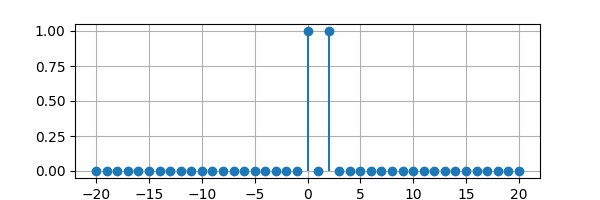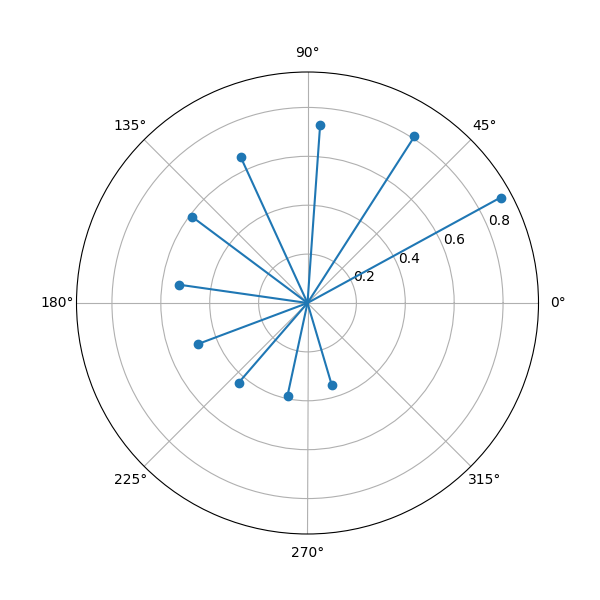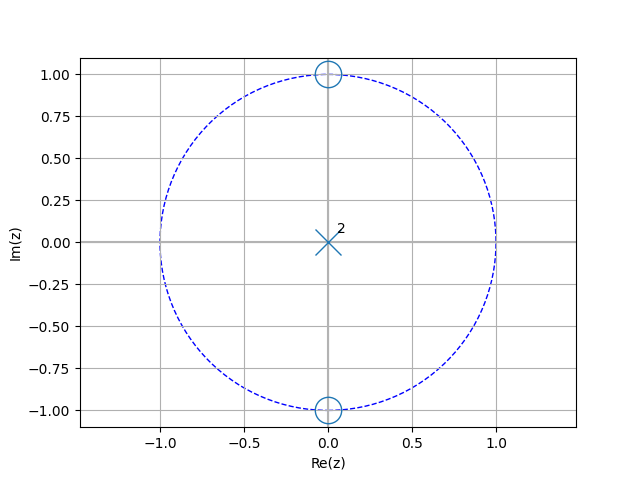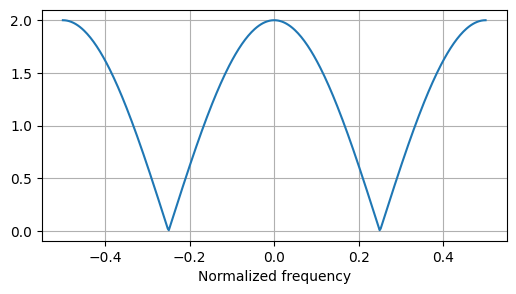# Discrete-time signals¶

There are a number of domain variables for discrete-time signals:

• n for discrete-time signals, for example, 3 * u(n - 2)

• f for linear frequency from a DTFT

• F for normalized linear frequency, F = f * dt

• k for discrete-frequency spectra

• omega for angular frequency from a DTFT, omega = 2 * pi * f

• z for z-transforms, for example, Y(z)

• Omega for normalized angular frequency from a DTFT, Omega = omega * dt

The n, k, and z variables share many of the attributes and methods of their continuous-time equivalents, t, f, and s, Expressions.

The discrete-time signal can be plotted using the plot() method. For example:

from lcapy import n, delta
from matplotlib.pyplot import savefig

x = delta(n) + delta(n - 2)
x.plot(figsize=(6, 2))

savefig('dt1-plot1.png')A complex discrete-time signal can be plotted in polar coordinates using the plot() method with the polar argument, for example:

from lcapy import j, n, exp
from matplotlib.pyplot import savefig

x = 0.9**n * exp(j * n * 0.5)
x.plot((1, 10), figsize=(6, 6), polar=True)

savefig('cdt1-plot1.png')## Functions¶

There are two special discrete time functions:

• delta(n) or ui(n) or UnitImpulse(n): the discrete unit impulse. This is one when n=0 and zero otherwise.

• u(n) or us(n) or UnitStep(n): the discrete unit step. This is one when n>=0 and zero otherwise.

## Sequences¶

Generic sequences can be created using the seq function. For example:

>>> s = seq((1, 2, 3))
{_1, 2, 3}


Note, the underscore marks the element in the sequence where n = 0. By default, seq() creates a discrete-time domain sequence. The domain can be specified with the domain argument. This can be either n, k, or z. For example, a Z-domain sequence is created with:

>>> s = seq((1, 2, 3), domain=z)
{_1, 2, 3}


Here’s an example where the sequence is specified as a string:

>>> s = seq('1, _2, 3')
{1, _2, 3}


Sequences can also be generated from a discrete-time expression, for example:

>>> x = delta(n) + 2 * delta(n - 2)
>>> seq = x.seq((-5, 5))
>>> seq
{0, 0, 0, 0, 0, _1, 0, 2, 0, 0, 0}


Note, the underscore marks the origin; the element in the sequence where n = 0.

Sequences can have quantities, for example, a discrete-time voltage sequence is created with:

>>> v = voltage(seq((1, 2, 3), domain=n))


The extent of a sequence is given by the extent attribute.

>>> seq.extent
>>> 3


Each element in a sequence has a sequence index. The sequence indices are return as a list by the n attribute. For example:

>>> x = seq('1, _2, 3, 4')
>>> x.n
[-1, 0, 1, 2]


The origin of a sequence is given by the origin attribute. This indicates the element index where n = 0. For example:

>>> x = seq('1, _2, 3, 4')
>>> x.origin
1
>>> x = seq('1, 2, _3, 4')
>>> x.origin
2


The origin can be changed:

>>> x = seq('1, 2, _3, 4')
>>> x.origin = 1
>>> x
{1, _2, 3, 4}


Specific elements in the sequence can be accessed using call notation:

>>> x = seq('1, _2, 3, 4')
>>> x(0)
2
>>> x(1)
3


Specific elements can also be accessed using array notation. Note, the argument specifies the element sequence index, for example:

>>> x = seq('1, _2, 3, 4')
>>> x
2
>>> x
3


If you want the first element convert the sequence to a list or ndarray, for example:

>>> x = seq('1, _2, 3, 4')
>>> array(x)
1

Sequences behave like lists and thus the + operator concatenates sequences::
>>> seq((1, 2, 3)) + seq('{4, 5}')
{_1, 2, 3, 4, 5}


Note, this ignores the origins.

Similarly, the * operator repeats sequences a specified number of times, for example:

>>> seq((1, 2, 3)) * 2
{_1, 2, 3, 1, 2, 3}


To add sequences element by element, it is necessary to explicitly convert each sequence to an array, add the arrays assuming they are equal length, and convert back to a sequence, for example:

>>> seq(seq((1, 2, 3)).as_array() + seq('{4, _5, 6}').as_array())
{_5, 7, 9}


Note, this ignores the origins.

Sequences can be convolved, for example:

>>> seq((1, 2, 3)).convolve(seq((1, 1))
{_1, 3, 5, 3}


Sequences can be evaluated and converted to a new sequence of floating point values using the evalf() method. This has an argument to specify the number of decimal places. For example:

>>> seq((pi, pi * 2))
{_π, 2⋅π}
>>> seq((pi, pi * 2)).evalf(3)
{_3.14, 6.28}


Sequences can be evaluated and converted to a NumPy array with the as_array() method:

>>> x = seq('1, _2, 3, 4')
>>> a = x.as_array()
>>> a
array([1., 2., 3., 4.])


Alternatively, the evaluate() method can be used to access and convert a single element or multiple elements. If the argument is a scalar, a real or complex Python scalar is returned. If the argument is iterable (tuple, list, ndarray), a NumPy real or complex ndarray is returned. Note, argument values outside the sequence return zero. Here is an example:

>>> x = seq('1, _2, 3, 4')
>>> x.evaluate(5)
0
>>> a = x.evaluate((1, 2))
>>> a
array([3., 4.])


Sequences can be converted to discrete-time domain or discrete-frequency domain expressions, for example:

>>> seq((1, 2)).expr
δ[n] + 2⋅δ[n - 2]


The discrete Fourier transform (DFT), inverse discrete Fourier transform (IDFT) z-transform (ZT), and inverse z-transform (IZT) can be performed using the DFT(), IDFT(), ZT(), and IZT() methods. In each case, a new sequence is returned. For example:

>>> seq((1, 2, 3, 4)).ZT()
⎧    2  3   4 ⎫
⎪_1, ─, ──, ──⎪
⎨    z   2   3⎬
⎪       z   z ⎪
⎩             ⎭

>>> seq((1, 2, 3, 4)).DFT()
{_10, -2 + 2⋅ⅉ, -2, -2 - 2⋅ⅉ}


### Sequence operators¶

Lcapy overloads the leftshift operator and the rightshift operator to shift sequences. For example:

>>> a = seq((1, 2, 3))
>>> a >> 2
{_0, 0, 1, 2, 3}
>>> a << 2
{1, 2, _3}


### Sequence attributes¶

• expr convert to a discrete-time or discrete-frequency expression

• extent the extent of the sequence

• n the sequence indices

• origin the element index for n = 0

• vals the sequence values as a list

### Sequence methods¶

These methods do not modify the sequence but return a new sequence, NumPy ndarray, or, Lcapy expression.

• as_array() convert to NumPy ndarray

• as_impulses() convert to a weighted sum of unit impulses expression

• convolve() convolve with another sequence

• delay() delay by an integer number of samples (the sequence is advanced if the argument is negative)

• DFT() compute discrete Fourier transform as a sequence

• DTFT() compute discrete-time Fourier transform

• evalf() convert each element in sequence to a SymPy floating-point value with a specified number of digits

• evaluate() evaluate sequence at specified indices and return as NumPy ndarray

• IDFT() compute inverse discrete Fourier transform as a sequence

• IZT() compute inverse z-transform as a sequence

• lfilter() filter by DLTI filter

• simplify() simplify each expression in sequence

• prune() remove zeroes from the ends of the sequence

• plot() plot sequence as a lollipop (stem) plot

• zeroextend() add zeroes at either start or end so origin is included

• ZT() compute z-transform as a sequence

## Discrete-time (n-domain) expressions¶

Lcapy refers to Discrete-time expressions as n-domain expressions. They are of class DiscreteTimeDomainExpression and can be created explicitly using the n-domain variable n. For example:

>>> 2 * u(n) + delta(n - 1)
2⋅u[n] + δ[n - 1]


In this expression u(n) denotes the unit step and delta(n) denotes the unit impulse. Square brackets are used in printing to reduce confusion with the Heaviside function and Dirac delta.

Discrete-time expressions can be converted to sequences using the seq() method. For example:

>>> (delta(n) + 2 * delta(n - 1) + 3 * delta(n - 3)).seq()
{_1, 2, 0, 3}


The seq() method has an argument to specify the extent of the sequence. This is required if the sequences have infinite extent. For example:

>>> (2 * u(n) + delta(n - 1)).seq((-10, 10))
{_2, 3, 2, 2, 2, 2, 2, 2, 2, 2}


In this example the zero samples have been removed but the sequence has been truncated.

The z-transform of a discrete-time expression can be found with the ZT() method:

>>> (delta(n) + 2 * delta(n - 2)).ZT()
2
1 + ──
2
z


A more compact notation is to pass z as an argument:

>>> (delta(n) + 2 * delta(n - 2))(z)
2
1 + ──
2
z


The discrete-time Fourier transform (DTFT) of a discrete-time expression can be found with the DTFT() method:

>>> (delta(n) + 2 * delta(n - 2)).DTFT()
-4⋅ⅉ⋅π⋅Δₜ⋅f
1 + 2⋅ℯ


A more compact notation is to pass f as an argument:

>>> (delta(n) + 2 * delta(n - 2))(f)
-4⋅ⅉ⋅π⋅Δₜ⋅f
1 + 2⋅ℯ


The discrete Fourier transform (DFT) converts a discrete-time expression to a discrete-frequency expression. This is performed using the DFT() method or using a k argument. For example:

>>> (delta(n) + 2 * delta(n - 2))(k)
-4⋅ⅉ⋅π⋅k
─────────
N
1 + 2⋅ℯ


If N is known, it can be specified as an argument. For example:

>>> (delta(n) + 2 * delta(n - 2))(k, N=4)
-ⅉ⋅π⋅k
1 + 2⋅ℯ


Evaluation of the DFT can be prevented by setting evaluate=False,

>>> (delta(n) + 2 * delta(n - 2))(k, N=4, evaluate=False)
N
____
╲
╲                        -2⋅ⅉ⋅π⋅k⋅n
╲                       ───────────
╱                            N
╱   (δ[n] + 2⋅δ[n - 2])⋅ℯ
╱
‾‾‾‾
n = 0


## Discrete-frequency (k-domain) expressions¶

Lcapy refers to discrete-frequency expressions as k-domain expressions. They are of class DiscreteFourierDomainExpression and can be created explicitly using the k-domain variable k. For example:

>>> 2 * u(k) + delta(k - 1)
2⋅u[k] + δ[k - 1]


Discrete-frequency expressions can be converted to sequences using the seq() method. For example:

>>> (delta(k) + 2 * delta(k - 1) + 3 * delta(k - 3)).seq()
{_1, 2, 0, 3}


## Z-domain expressions¶

Z-domain expressions can be constructed using the z-domain variable z, for example:

>>> 1 + 1 / z
1
1 + ─
z


Alternatively, they can be generated using a z-transform of a discrete-time signal.

Z-domain expressions are objects of the ZDomainExpression class. They are functions of the complex variable z and are similar to LaplaceDomainExpression objects. The general form of a z-domain expression is a rational function so all the s-domain formatting methods are applicable (see Formatting methods).

The poles and zeros of a z-domain expression can be plotted using the plot() method. For example:

from lcapy import delta
from lcapy.discretetime import n, z
from matplotlib.pyplot import savefig

x = delta(n) + delta(n - 2)
X = x(z)
X.plot()

savefig('dt1-pole-zero-plot1.png')## Transforms¶

Lcapy implements a number of transforms for converting between different domains. The explicit methods are:

• DFT() Discrete Fourier transform

• DTFT() Discrete-time Fourier transform

• ZT() Z-transform

• IDFT() Inverse discrete Fourier transform

• IDTFT() Inverse discrete-time Fourier transform

• IZT() Inverse z-transform

### Z-transform (ZT)¶

Lcapy uses the unilateral z-transform, defined as:

$X(z) = \sum_{n=0}^{\infty} x(n) z^{-n}$

The z-transform is performed explicitly with the ZT() method:

>>> x = delta(n) + 2 * delta(n - 2)
>>> x.ZT()
>>>      2
1 + ──
2
z


It is also performed implicitly with z as an argument:

>>> x(z)
>>>     2
1 + ──
2
z


### Inverse z-transform (IZT)¶

The inverse unilateral z-transform is not unique and is only defined for $$n \ge 0$$. For example:

>>> H = z / (z - 'a')
>>> H(n)
⎧ n
⎨a   for n ≥ 0
⎩


If the result is known to be causal, then use:

>>> H(n, causal=True)
n
a ⋅u(n)


### Discrete time Fourier transform (DTFT)¶

The DTFT converts an n-domain or z-domain expression into the f-domain (continuous Fourier domain). Note, unlike the Fourier transform, this is periodic with period $$1/\Delta t$$. It is defined by

$X_{\frac{1}{\Delta t}}(f) = \sum_{n=-\infty}^{\infty} x(n) e^{-2 \mathrm{j} \pi n \Delta t f}$

If $$x(n)$$ is the impulse response of a causal and stable DLTI system, the DTFT can be found by substituting $$z = \exp(-2 \mathrm{j} \pi \Delta t f)$$ into the z-transform of $$x(n)$$.

Here is an example:

>>> sign(n).DTFT()
2
────────────────
-2⋅ⅉ⋅π⋅Δₜ⋅f
1 - ℯ


Alternatively, the transform can be invoked using f as an argument:

>>> sign(n)(f)
2
────────────────
-2⋅ⅉ⋅π⋅Δₜ⋅f
1 - ℯ


Here’s an example of plotting the DTFT:

from lcapy import delta
from lcapy.discretetime import n, dt
from matplotlib.pyplot import savefig

x = delta(n) + delta(n - 2)
abs(x.DTFT().subs(dt, 1)).plot(norm=True, figsize=(6, 3))

savefig('dt1-DTFT-plot1.png', bbox_inches='tight')The DTFT can be confusing due to the number of definitions commonly used. Due to the periodicity it is common to define a normalized frequency $$F = f \Delta t$$ and so

$X_1(F) = \sum_{n=-\infty}^{\infty} x(n) e^{-2 \mathrm{j} \pi n F}$

Here is an example:

>>> sign(n).DTFT(F)
2
─────────────
-2⋅ⅉ⋅π⋅F
1 - ℯ


Alternatively, the transform can be invoked using F as an argument:

>>> sign(n)(F)
2
─────────────
-2⋅ⅉ⋅π⋅F
1 - ℯ


Another option is to use normalized angular frequency $$\Omega = 2\pi f \Delta t$$

$X_{2\pi}(\Omega) = \sum_{n=-\infty}^{\infty} x(n) e^{-\mathrm{j} n \Omega}$

Here is an example:

>>> sign(n).DTFT(Omega)
2
────────────────
-2⋅ⅉ⋅π⋅Δₜ⋅f
1 - ℯ


Alternatively, the transform can be invoked using Omega as an argument:

>>> sign(n)(Omega)
2
────────────────
-2⋅ⅉ⋅π⋅Δₜ⋅f
1 - ℯ


A normalized discrete-time angular Fourier transform of x(n) can be plotted as follows:

>>> x.DTFT(Omega).plot()


This plots the normalized angular frequency between $$-\pi$$ and $$\pi$$.

The DTFT, $$X_{\frac{1}{\Delta t}}(f)$$, is related to the Fourier transform, $$X(f)$$, by

$X_{\frac{1}{\Delta t}}(f) = \frac{1}{\Delta t} \sum_{m=-\infty}^{\infty} X\left(f-\frac{m}{\Delta t}\right)$

Note, some definitions do not include the scale factor $$1 / \Delta t$$ since it assumed that $$x(n) = \Delta t x(n \Delta t)$$. However, this introduces units confusion.

The DTFT is periodic in frequency with a period $$1 / \Delta t$$ and provided the signal is not aliased, all the information about the signal can be obtained from any frequency range of interval $$1 / \Delta t$$.

By default Lcapy returns an expression showing the infinite number of spectral images. For example,

>>> nexpr(1).DTFT()
∞
____
╲
╲
╲    ⎛    m ⎞
╱   δ⎜f - ──⎟
╱     ⎝    Δₜ⎠
╱
‾‾‾‾
m = -∞
────────────────
Δₜ


All the images can be removed with the remove_images() method. For example:

>>> nexpr(1).DTFT().remove_images()
δ(f)
────
Δₜ


Alternatively, the images argument can be used with the DTFT() method:

>>> nexpr(1).DTFT(images=0)
δ(f)
────
Δₜ


The number of images can be specified with the m1 and m2 arguments to the remove_images() method. This is useful for plotting. For example,

>>> nexpr(1).DTFT(F).remove_images(-2, 2).doit()
δ(F) + δ(F - 2) + δ(F - 1) + δ(F + 1) + δ(F + 2)


### Inverse discrete-time Fourier transform (IDTFT)¶

Like the DTFT, the IDFT has many commonly used definitions. In terms of linear frequency,

$x(n) = \Delta t \int_{-\frac{1}{2\Delta t}}^{\frac{1}{2\Delta t}} X_{\frac{1}{\Delta t}}(f) e^{2 \mathrm{j} \pi n \Delta t f} \mathrm{d}f$

where $$x(n)$$ denotes $$x(n \Delta t)$$.

In terms of normalized linear frequency,

$x(n) = \int_{-\frac{1}{2}}^{\frac{1}{2}} X_{1}(f) e^{2 \mathrm{j} \pi n F} \mathrm{d}F$

In terms of normalized angular frequency,

$x(n) = \frac{1}{2\pi} \int_{-\pi}^{\pi} X_{2\pi}(f) e^{\mathrm{j} n \Omega} \mathrm{d}\Omega$

### Discrete Fourier transform (DFT)¶

The DFT converts an n-domain expression to a k-domain expression. The definition used by Lcapy is:

$X(k) = \sum_{k=0}^{N - 1} x(n) e^{\frac{-\mathrm{j} 2\pi k n}{N}}$

Note, both $$x(n)$$ and $$X(k)$$ are assumed to periodic with period $$N$$, i.e., $$x(n + m N) = x(n)$$ for integer $$m$$.

### Inverse discrete Fourier transform (IDFT)¶

The IDFT converts a k-domain expression to an n-domain expression. The definition used by Lcapy is:

$x(n) = \frac{1}{N} \sum_{k=0}^{N - 1} X(k) e^{\frac{\mathrm{j} 2 \pi k n}{N}}$

Again, both $$x(n)$$ and $$X(k)$$ are assumed to periodic with period $$N$$, i.e., $$x(n + m N) = x(n)$$ for integer $$m$$.

### Bilinear transform¶

The bilinear transform can be used to approximate an s-domain expression with a z-domain expression using $$s \approx \frac{2}{\Delta t} \frac{1 - z^{-1}}{1 + z^{-1}}$$ (see Discrete-time approximation for other methods). This is performed by the bilinear_transform() method of s-domain objects, for example:

>>> H = s / (s - 'a')
>>> Hz = H.bilinear_transform().simplify()
>>> Hz
2⋅(1 - z)
──────────────────────
Δₜ⋅a⋅(z + 1) - 2⋅z + 2


The related method inverse_bilinear_transform() converts an s-domain expression to the z-domain using $$z \approx (1 + 0.5 \Delta t s) / (1 - 0.5 \Delta t s)$$.

Here’s an example of the bilinear transform applied for an RC low-pass filter.

>>> from lcapy import Circuit, s, t
>>> net = Circuit("""
R 1 2; right
W 0 0_2; right
C 2 0_2; down
W 2 3; right=0.5
W 0_2 0_3; right=0.5""")


This has a transfer function:

>>> H = net.transfer(1, 0, 3, 0)
>>> H
1
─────────────
⎛     1 ⎞
C⋅R⋅⎜s + ───⎟
⎝    C⋅R⎠


and an impulse response:

>>> H(t)
-t
───
C⋅R
e   ⋅u(t)
─────────
C⋅R


Using the bilinear transform, the discrete-time transfer function is

>>> H.bilinear_transform().canonical()
Δₜ⋅(z + 1)
──────────────────────────────
⎛    -2⋅C⋅R + Δₜ⎞
⎜z + ───────────⎟⋅(2⋅C⋅R + Δₜ)
⎝     2⋅C⋅R + Δₜ⎠


with a discrete-time impulse response

>>> from lcapy.discretetime import n
>>> H.bilinear_transform()(n).simplify()
⎛                  n                         ⎞
⎜      ⎛2⋅C⋅R - Δₜ⎞                          ⎟
Δₜ⋅⎜4⋅C⋅R⋅⎜──────────⎟ ⋅u(n) - (2⋅C⋅R + Δₜ)⋅δ[n]⎟
⎝      ⎝2⋅C⋅R + Δₜ⎠                          ⎠
─────────────────────────────────────────────────
(2⋅C⋅R - Δₜ)⋅(2⋅C⋅R + Δₜ)